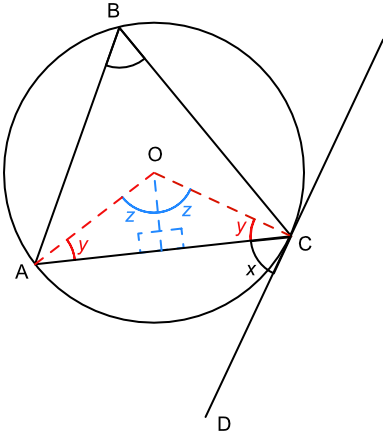Circle Theorems - Alternate Segments

## Circle Theorems - Alternate Segments

An angle between the tangent and the chord is therefore equal to the angle in the alternate segment.

The alternate angle is the angle made in the other sector from a chord. In the example below, the alternate angle to ∠ACD (shown as x) is the angle ∠ABC - it is on the other side of the chord.

Note: you do not need to know how to prove this, but you do need to know the theorem and how to use it.

DC is a tangent to the circle. A triangle ∠ABC has points A, B and C on the circumference of a circle with centre O.

Let the angle ∠ACD be x. Add a triangle AOC with the angle ∠OCA as y.

A tangent is at right angles to the radius; therefore x + y = 90º

Bisect the triangle ∠AOC from the centre: the triangle is an Isosceles triangle and the bisecting line meets the chord at a right angle. Let the bisected angle have a value of z.

Angles in a triangle add to 180º; z + y + 90 = 180, or z + y = 90º.

Match the two equations: x + y = z + y therefore x = z

The angle at the centre is twice the value of the angle at the circumference when subtended by the same arc: ∠AOC = 2z therefore ∠ABC = z = x.## Example 1

ED is a tangent to a circle, touching at point A. Triangle ABC is a triangle proscribed within the circle. Angle ∠DAC has a value of 59º. What is the value of angle ∠ABC?Alternate Segment: the angle between the tangent and the chord is equal to the value of the angle in the alternate segment.